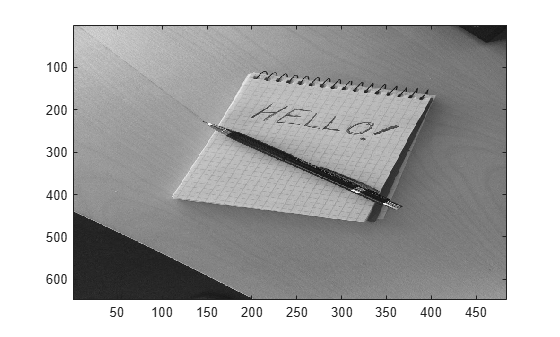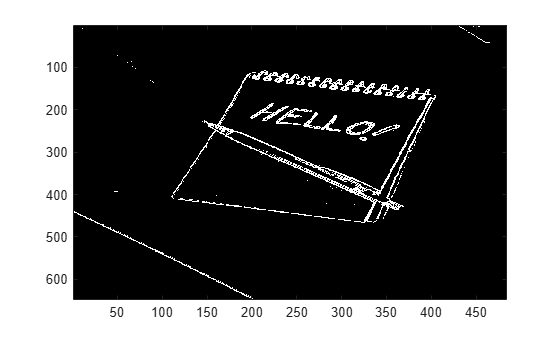# Generate Code to Detect Edges on Images

This example shows how to generate a standalone C library from MATLAB® code that implements a simple Sobel filter that performs edge detection on images. The example also shows how to generate and test a MEX function in MATLAB prior to generating C code to verify that the MATLAB code is suitable for code generation.

### About the `sobel` Function

The `sobel.m` function takes an image (represented as a double matrix) and a threshold value and returns an image with the edges detected (based on the threshold value).

`type sobel`
```% edgeImage = sobel(originalImage, threshold) % Sobel edge detection. Given a normalized image (with double values) % return an image where the edges are detected w.r.t. threshold value. function edgeImage = sobel(originalImage, threshold) %#codegen assert(all(size(originalImage) <= [1024 1024])); assert(isa(originalImage, 'double')); assert(isa(threshold, 'double')); k = [1 2 1; 0 0 0; -1 -2 -1]; H = conv2(double(originalImage),k, 'same'); V = conv2(double(originalImage),k','same'); E = sqrt(H.*H + V.*V); edgeImage = uint8((E > threshold) * 255); ```

### Generate the MEX Function

Generate a MEX function using the `codegen` command.

`codegen sobel`
```Code generation successful. ```

Before generating C code, you should first test the MEX function in MATLAB to ensure that it is functionally equivalent to the original MATLAB code and that no run-time errors occur. By default, `codegen` generates a MEX function named `sobel_mex` in the current folder. This allows you to test the MATLAB code and MEX function and compare the results.

### Read in the Original Image

Use the standard `imread` command.

```im = imread('hello.jpg'); image(im);```### Convert Image to a Grayscale Version

Convert the color image (shown above) to an equivalent grayscale image with normalized values (0.0 for black, 1.0 for white).

`gray = (0.2989 * double(im(:,:,1)) + 0.5870 * double(im(:,:,2)) + 0.1140 * double(im(:,:,3)))/255;`

### Run the MEX Function (The Sobel Filter)

Pass the normalized image and a threshold value.

`edgeIm = sobel_mex(gray, 0.7);`

### Display the Result

```im3 = repmat(edgeIm, [1 1 3]); image(im3);```### Generate Standalone C Code

`codegen -config coder.config('lib') sobel`
```Code generation successful. ```

Using `codegen` with the `-config coder.config('lib')` option produces a standalone C library. By default, the code generated for the library is in the folder `codegen/lib/sobel/`.

### Inspect the Generated Function

`type codegen/lib/sobel/sobel.c`
```/* * File: sobel.c * * MATLAB Coder version : 5.2 * C/C++ source code generated on : 23-Feb-2021 13:37:12 */ /* Include Files */ #include "sobel.h" #include "conv2AXPYSameCMP.h" #include "sobel_data.h" #include "sobel_emxutil.h" #include "sobel_initialize.h" #include "sobel_types.h" #include <math.h> /* Function Definitions */ /* * Arguments : const emxArray_real_T *originalImage * double threshold * emxArray_uint8_T *edgeImage * Return Type : void */ void sobel(const emxArray_real_T *originalImage, double threshold, emxArray_uint8_T *edgeImage) { emxArray_real_T *H; emxArray_real_T *V; int k; int nx; if (!isInitialized_sobel) { sobel_initialize(); } emxInit_real_T(&H, 2); emxInit_real_T(&V, 2); /* edgeImage = sobel(originalImage, threshold) */ /* Sobel edge detection. Given a normalized image (with double values) */ /* return an image where the edges are detected w.r.t. threshold value. */ conv2AXPYSameCMP(originalImage, H); b_conv2AXPYSameCMP(originalImage, V); nx = H->size * H->size; for (k = 0; k < nx; k++) { H->data[k] = H->data[k] * H->data[k] + V->data[k] * V->data[k]; } emxFree_real_T(&V); nx = H->size * H->size; for (k = 0; k < nx; k++) { H->data[k] = sqrt(H->data[k]); } k = edgeImage->size * edgeImage->size; edgeImage->size = H->size; edgeImage->size = H->size; emxEnsureCapacity_uint8_T(edgeImage, k); nx = H->size * H->size; for (k = 0; k < nx; k++) { edgeImage->data[k] = (unsigned char)((H->data[k] > threshold) * 255U); } emxFree_real_T(&H); } /* * File trailer for sobel.c * * [EOF] */ ```

## Support평가판 신청